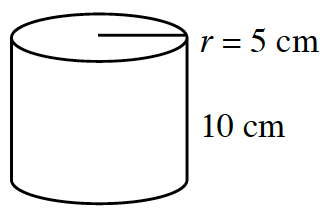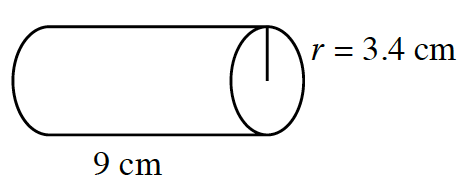### Home > CC2MN > Chapter 11 > Lesson 11.1.2 > Problem11-26

11-26.

Find the area of the base of each cylinder below, and then calculate the volume of each cylinder. Show your steps.

1.The base is a circle, so find the area of the circle using the following equation:
$\text{Area} = π(5^2)$

$\text{Area} = π(5^2)$

$\text{Area} = 25π \text{ cm}^2$ or approximately $78.54 \text{ cm}^2$.
Now use the equation for the volume of a cylinder:
$\text{Volume = (area of base) (height)}$

$\text{Volume} = (78.54)(10)$

$785.4 \text{ cm}^3$

1.See part (a).